# Visualizing Earnings Based On College Majors¶

Dataset Description : We'll be working with a dataset on the job outcomes of students who graduated from college between 2010 and 2012. The original data on job outcomes was released by American Community Survey, which conducts surveys and aggregates the data. FiveThirtyEight cleaned the dataset and released it on their Github repo.

Attribute description : Each row in the dataset represents a different major in college and contains information on gender diversity, employment rates, median salaries, and more. Here are some of the columns in the dataset:

Row Description
Rank Rank by median earnings (the dataset is ordered by this column)
Major_code Major code
Major Major description.
Major_category Category of major.
Total Total number of people with major.
Sample_size Sample size (unweighted) of full-time.
Men Male graduates.
Women Female graduates.
ShareWomen Women as share of total.
Employed Number employed.
Median Median salary of full-time, year-round workers.
Low_wage_jobs Number in low-wage service jobs.
Full_time Number employed 35 hours or more.
Part_time Number employed less than 35 hours.

## Aim :¶

1. Using 'scatter plots' we aim to determine : Do students in more popular majors make more money?
2. Using 'histograms' we aim to determine : How many majors are predominantly male or female?
3. Using 'bar plots' we aim to determine : Which category of majors have the most students?

## Dataset cleaning :¶

Let's clean the dataset before performing any analysis so that we can extract clean and precise inferences from our dataset inspection.

In :
# importing the required libraries:
import pandas as pd
import numpy as np
import matplotlib.pyplot as plt

# in order to display the plot inline
%matplotlib inline

In :
# reading and importing the dataset into a onadas dataframe:

Out:
Rank                                        1
Major_code                               2419
Major                   PETROLEUM ENGINEERING
Total                                    2339
Men                                      2057
Women                                     282
Major_category                    Engineering
ShareWomen                           0.120564
Sample_size                                36
Employed                                 1976
Full_time                                1849
Part_time                                 270
Full_time_year_round                     1207
Unemployed                                 37
Unemployment_rate                   0.0183805
Median                                 110000
P25th                                   95000
P75th                                  125000
College_jobs                             1534
Non_college_jobs                          364
Low_wage_jobs                             193
Name: 0, dtype: object
In :
# Let's see how the data is structured :

Out:
Rank Major_code Major Total Men Women Major_category ShareWomen Sample_size Employed ... Part_time Full_time_year_round Unemployed Unemployment_rate Median P25th P75th College_jobs Non_college_jobs Low_wage_jobs
0 1 2419 PETROLEUM ENGINEERING 2339.0 2057.0 282.0 Engineering 0.120564 36 1976 ... 270 1207 37 0.018381 110000 95000 125000 1534 364 193
1 2 2416 MINING AND MINERAL ENGINEERING 756.0 679.0 77.0 Engineering 0.101852 7 640 ... 170 388 85 0.117241 75000 55000 90000 350 257 50
2 3 2415 METALLURGICAL ENGINEERING 856.0 725.0 131.0 Engineering 0.153037 3 648 ... 133 340 16 0.024096 73000 50000 105000 456 176 0
3 4 2417 NAVAL ARCHITECTURE AND MARINE ENGINEERING 1258.0 1123.0 135.0 Engineering 0.107313 16 758 ... 150 692 40 0.050125 70000 43000 80000 529 102 0
4 5 2405 CHEMICAL ENGINEERING 32260.0 21239.0 11021.0 Engineering 0.341631 289 25694 ... 5180 16697 1672 0.061098 65000 50000 75000 18314 4440 972

5 rows × 21 columns

In :
# Let's get the summary of all the numeric columns in the dataset:

Out:
Rank Major_code Total Men Women ShareWomen Sample_size Employed Full_time Part_time Full_time_year_round Unemployed Unemployment_rate Median P25th P75th College_jobs Non_college_jobs Low_wage_jobs
count 173.000000 173.000000 172.000000 172.000000 172.000000 172.000000 173.000000 173.000000 173.000000 173.000000 173.000000 173.000000 173.000000 173.000000 173.000000 173.000000 173.000000 173.000000 173.000000
mean 87.000000 3879.815029 39370.081395 16723.406977 22646.674419 0.522223 356.080925 31192.763006 26029.306358 8832.398844 19694.427746 2416.329480 0.068191 40151.445087 29501.445087 51494.219653 12322.635838 13284.497110 3859.017341
std 50.084928 1687.753140 63483.491009 28122.433474 41057.330740 0.231205 618.361022 50675.002241 42869.655092 14648.179473 33160.941514 4112.803148 0.030331 11470.181802 9166.005235 14906.279740 21299.868863 23789.655363 6944.998579
min 1.000000 1100.000000 124.000000 119.000000 0.000000 0.000000 2.000000 0.000000 111.000000 0.000000 111.000000 0.000000 0.000000 22000.000000 18500.000000 22000.000000 0.000000 0.000000 0.000000
25% 44.000000 2403.000000 4549.750000 2177.500000 1778.250000 0.336026 39.000000 3608.000000 3154.000000 1030.000000 2453.000000 304.000000 0.050306 33000.000000 24000.000000 42000.000000 1675.000000 1591.000000 340.000000
50% 87.000000 3608.000000 15104.000000 5434.000000 8386.500000 0.534024 130.000000 11797.000000 10048.000000 3299.000000 7413.000000 893.000000 0.067961 36000.000000 27000.000000 47000.000000 4390.000000 4595.000000 1231.000000
75% 130.000000 5503.000000 38909.750000 14631.000000 22553.750000 0.703299 338.000000 31433.000000 25147.000000 9948.000000 16891.000000 2393.000000 0.087557 45000.000000 33000.000000 60000.000000 14444.000000 11783.000000 3466.000000
max 173.000000 6403.000000 393735.000000 173809.000000 307087.000000 0.968954 4212.000000 307933.000000 251540.000000 115172.000000 199897.000000 28169.000000 0.177226 110000.000000 95000.000000 125000.000000 151643.000000 148395.000000 48207.000000
In :
# Let's get some info about the datatypes too :

<class 'pandas.core.frame.DataFrame'>
RangeIndex: 173 entries, 0 to 172
Data columns (total 21 columns):
Rank                    173 non-null int64
Major_code              173 non-null int64
Major                   173 non-null object
Total                   172 non-null float64
Men                     172 non-null float64
Women                   172 non-null float64
Major_category          173 non-null object
ShareWomen              172 non-null float64
Sample_size             173 non-null int64
Employed                173 non-null int64
Full_time               173 non-null int64
Part_time               173 non-null int64
Full_time_year_round    173 non-null int64
Unemployed              173 non-null int64
Unemployment_rate       173 non-null float64
Median                  173 non-null int64
P25th                   173 non-null int64
P75th                   173 non-null int64
College_jobs            173 non-null int64
Non_college_jobs        173 non-null int64
Low_wage_jobs           173 non-null int64
dtypes: float64(5), int64(14), object(2)
memory usage: 28.5+ KB


We will drop all the rows with missing values. Matplotlib expects that columns of values we pass in have matching lengths and missing values will cause matplotlib to throw errors.

In :
# let's find all the missing values in the dataset:

print ("The number of rows containing empty values were : {}" .format(raw_data_count-cleaned_data_count))

The number of rows containing empty values were : 1


We found that there was only one row containing empty values and that was dropped. Now that the dataset is cleaned let's try to find some relations betweeen the various attributes of our dataset.

### 1. Using scatterplots to find the correlation between dataset attributes:¶

In :
# 1. between 'sample_size' and 'employed':
kind = 'scatter',
title = 'Employed vs. Sample_size',
xlim = (0),
ylim = (0)
)
print("The correlation value between the attibutes are : {}".

The correlation value between the attibutes are : 0.9644061612735608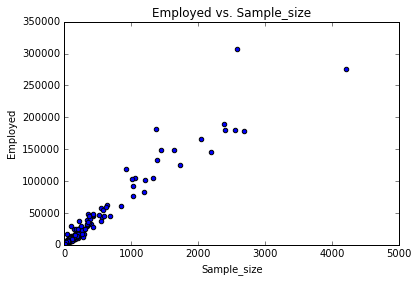We see a strong positive correlation between the attributes.

In :
# 2. between 'sample_size' and 'median':
kind = 'scatter',
title = 'Sample_size vs. Median',
xlim = 0,
ylim = 0
)
print("The correlation value between the attibutes are : {}"

The correlation value between the attibutes are : -0.06447499542762185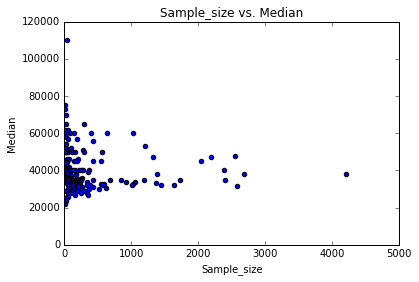Hence, we see a negative realtion between sample_size and median values in the dataset.

In :
# 3. Between 'Full_time' and 'Median':
kind = 'scatter',
title = 'Full_time vs. Median',
xlim = 0,
ylim = 0
)
print("The correlation value between the attibutes are : {}".

The correlation value between the attibutes are : -0.07903093567520722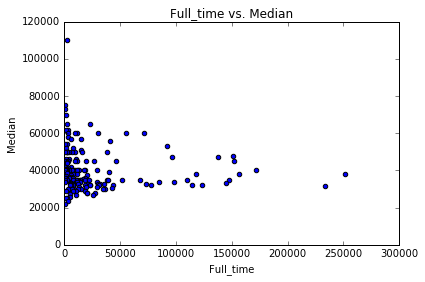We see a negative realtion between Full_time and median values in the dataset.

In :
# 3. Between 'ShareWomen' and 'Unemployment_rate':
kind = 'scatter',
title = 'ShareWomen vs. Unemployment_rate',
xlim = 0,
ylim = 0
)
print("The correlation value between the attibutes are : {}".

The correlation value between the attibutes are : 0.0732045786722932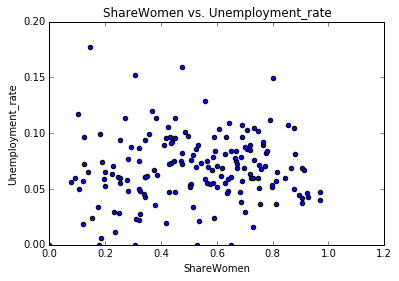We see a higly weak correlation between the values.

In :
# 4. Between 'Men' and 'Median':
kind = 'scatter',
title = 'Men vs. Median',
xlim = 0,
ylim = 0
)
print("The correlation value between the attibutes are : {}".

The correlation value between the attibutes are : 0.025990597811967213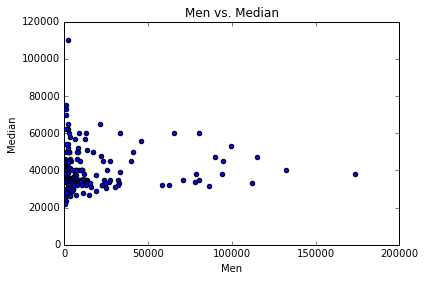We again witness a very weak correlation between the given attributes.

In :
# 5. Between 'Women' and 'Median':
kind = 'scatter',
title = 'Women vs. Median',
xlim = 0,
ylim = 0
)
print("The correlation value between the attibutes are : {}".

The correlation value between the attibutes are : -0.18284186159966848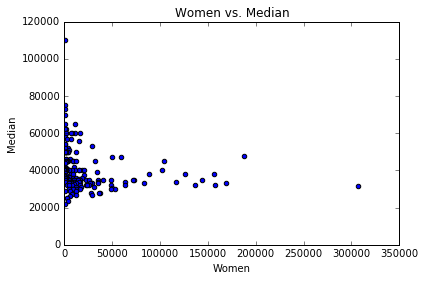We see a negative correlation between the attributes.

In :
# 6.between 'sample_size' and 'Unemployment_rate':
kind = 'scatter',
title = 'Sample_size vs. Unemployment_rate',
xlim = 0,
ylim = 0
)
print("The correlation value between the attibutes are : {}"

The correlation value between the attibutes are : 0.06295493699511233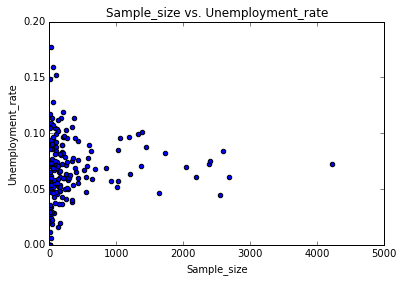We see a weak correlation between the attributes, similar to that between ShareWomen and Unemployment_rate.

In :
# 7.between 'ShareWmen' and 'Median':
kind = 'scatter',
title = 'ShareWomen vs. Median',
xlim = 0,
ylim = 0
)
print("The correlation value between the attibutes are : {}"

The correlation value between the attibutes are : -0.6186897512131612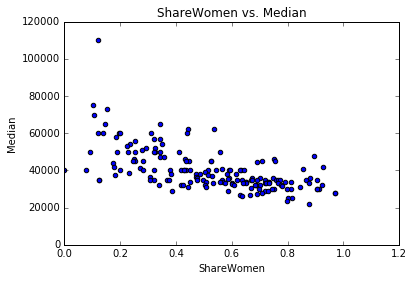We see a strong neegative correlation between 'ShareWomen' and'Median' attributes showing that : As the the percentage share of women increases, the median salary decreases.

### 2. Using histograms for futher exploration of the dataset attributes:¶

Let's generate histograms for columns in the dataset so as to explore their distributions.

In :
# Let's make a list of all the columns we need to make plot the histogram for:
cols_hist = ['Sample_size', 'Median' , 'Employed', 'Full_time', 'ShareWomen', 'Unemployment_rate',
'Men', 'Women']

In :
# Plotting the histograms using a loop:

# 1. 'Sample_size' :
recent_grads['Sample_size'].hist(bins= 20, range = (0, 5500) )
plt.title('Distribution of Sample_size')
plt.show()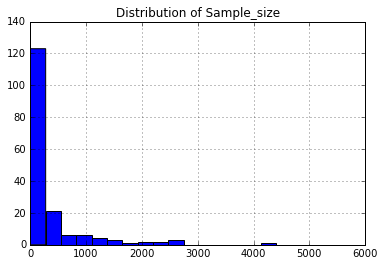In :
# 2. 'Median' :
plt.title('Distribution of Median')
plt.show()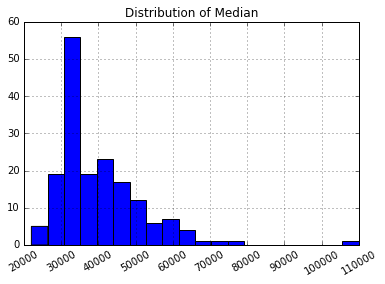In :
# 2. 'Employed' :
recent_grads['Employed'].hist(bins= 20, xrot= 30, range = (0, 350000))
plt.title('Distribution of Employed')
plt.show()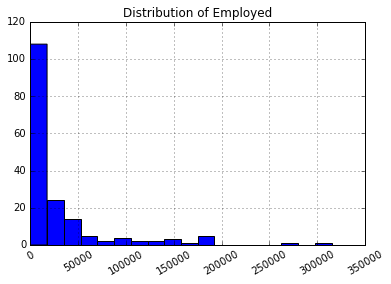In :
# 3. 'Full_time' :
plt.title('Distribution of Full_time')
plt.show()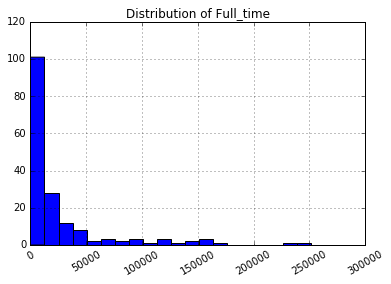In :
# 4. 'ShareWomen'
plt.title('Distribution of ShareWomen')
plt.show()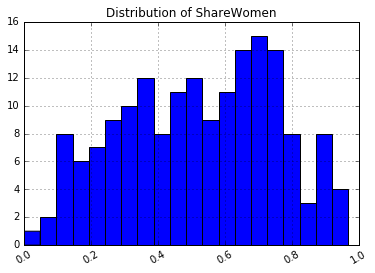In :
# 5. 'Unemployment_rate'
plt.title('Distribution of Unemployment_rate')
plt.show()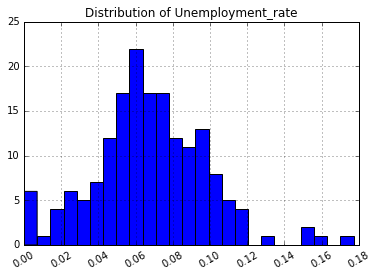In :
# 6. 'Men'
plt.title('Distribution of Men')
plt.show()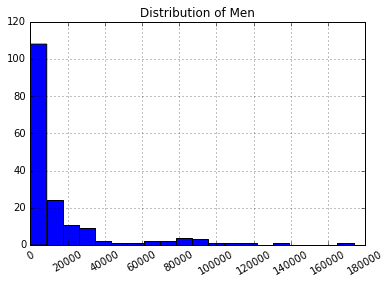In :
# 7. 'Women'
plt.title('Distribution of Women')
plt.show()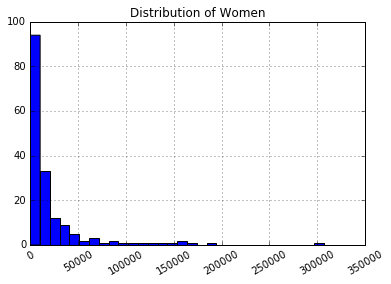### 3. Using scatter-matrix to find the correlation between dataset attributes:¶

A scatter matrix plot combines both scatter plots and histograms into one grid of plots and allows us to explore potential relationships and distributions simultaneously. A scatter matrix plot consists of n by n plots on a grid, where n is the number of columns, the plots on the diagonal are histograms, and the non-diagonal plots are scatter plots.

In :
# 1. plotting 'Sample_size' and'Median' using scatter_matrix plots:

Out:
array([[<matplotlib.axes._subplots.AxesSubplot object at 0x7f4b8e4a3208>,
<matplotlib.axes._subplots.AxesSubplot object at 0x7f4b8e4397f0>],
[<matplotlib.axes._subplots.AxesSubplot object at 0x7f4b8e383ac8>,
<matplotlib.axes._subplots.AxesSubplot object at 0x7f4b8e3be3c8>]],
dtype=object)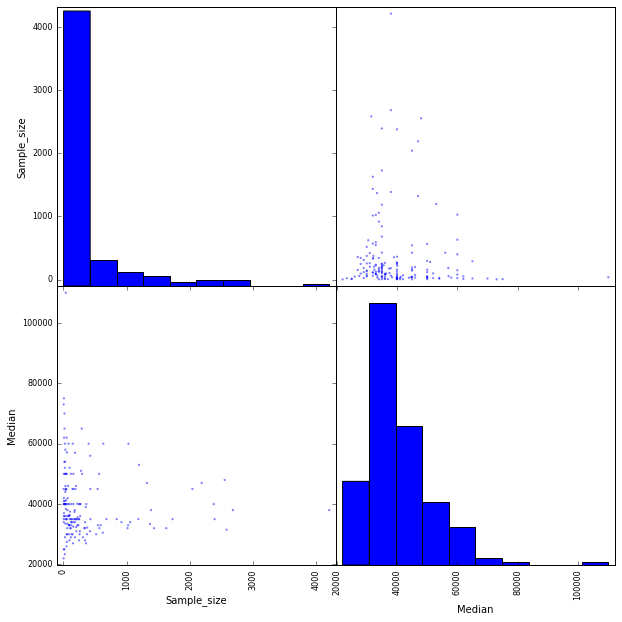In :
# 2.Let's plot a scatter matrix plot between sharewmen and low wage jobs:
pd.plotting.scatter_matrix(recent_grads[['ShareWomen', 'Low_wage_jobs', 'Unemployment_rate']], figsize= (10, 10))

Out:
array([[<matplotlib.axes._subplots.AxesSubplot object at 0x7f4b8e2e9080>,
<matplotlib.axes._subplots.AxesSubplot object at 0x7f4b8e255ef0>,
<matplotlib.axes._subplots.AxesSubplot object at 0x7f4b8e224828>],
[<matplotlib.axes._subplots.AxesSubplot object at 0x7f4b8e4df5f8>,
<matplotlib.axes._subplots.AxesSubplot object at 0x7f4b8e441f60>,
<matplotlib.axes._subplots.AxesSubplot object at 0x7f4b8e5445c0>],
[<matplotlib.axes._subplots.AxesSubplot object at 0x7f4b8e521c50>,
<matplotlib.axes._subplots.AxesSubplot object at 0x7f4b8e5eb128>,
<matplotlib.axes._subplots.AxesSubplot object at 0x7f4b8e7981d0>]],
dtype=object)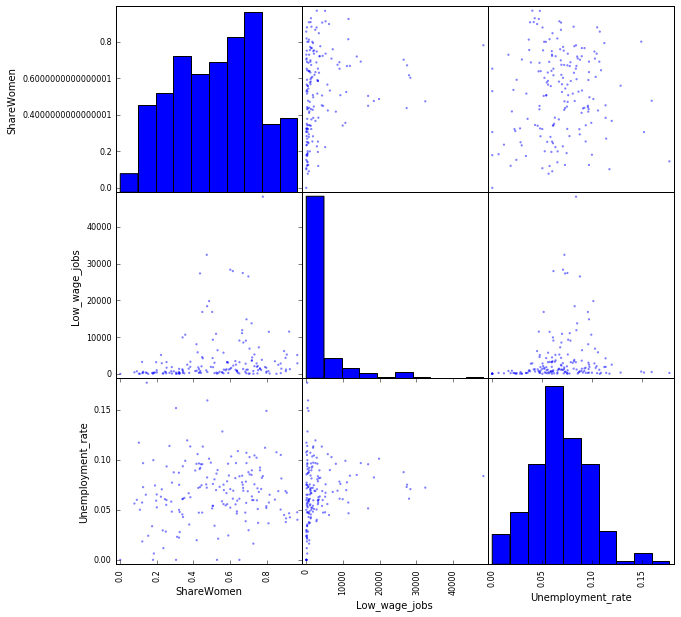In :
# 3. creating a 3*3 scatter matrix plot:
pd.plotting.scatter_matrix(recent_grads[['Sample_size', 'Median', 'Unemployment_rate']], figsize= (10, 10))

Out:
array([[<matplotlib.axes._subplots.AxesSubplot object at 0x7f4b8fa5ada0>,
<matplotlib.axes._subplots.AxesSubplot object at 0x7f4b9480c9e8>,
<matplotlib.axes._subplots.AxesSubplot object at 0x7f4b94407470>],
[<matplotlib.axes._subplots.AxesSubplot object at 0x7f4b9441b2e8>,
<matplotlib.axes._subplots.AxesSubplot object at 0x7f4b944d87b8>,
<matplotlib.axes._subplots.AxesSubplot object at 0x7f4b8fc90550>],
[<matplotlib.axes._subplots.AxesSubplot object at 0x7f4b940fa518>,
<matplotlib.axes._subplots.AxesSubplot object at 0x7f4b9480a128>,
<matplotlib.axes._subplots.AxesSubplot object at 0x7f4b94b0ab70>]],
dtype=object)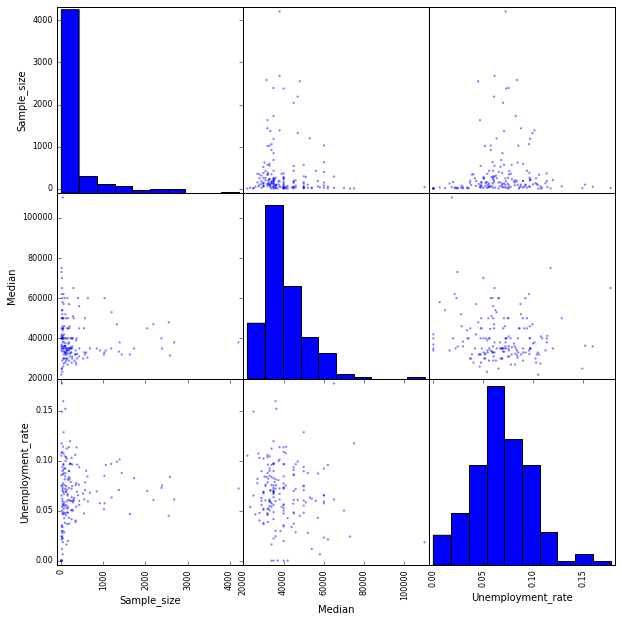In :
 # 4. creating a 3*3 scatter matrix plot:
pd.plotting.scatter_matrix(recent_grads[['ShareWomen', 'Median', 'Unemployment_rate']], figsize= (10, 10))

Out:
array([[<matplotlib.axes._subplots.AxesSubplot object at 0x7f4b8f186358>,
<matplotlib.axes._subplots.AxesSubplot object at 0x7f4b8efbeac8>,
<matplotlib.axes._subplots.AxesSubplot object at 0x7f4b8fb9f390>],
[<matplotlib.axes._subplots.AxesSubplot object at 0x7f4b94b07048>,
<matplotlib.axes._subplots.AxesSubplot object at 0x7f4b8e5cb278>,
<matplotlib.axes._subplots.AxesSubplot object at 0x7f4b8fb7d710>],
[<matplotlib.axes._subplots.AxesSubplot object at 0x7f4b8e686e10>,
<matplotlib.axes._subplots.AxesSubplot object at 0x7f4b8ed1d550>,
<matplotlib.axes._subplots.AxesSubplot object at 0x7f4b8ed41898>]],
dtype=object)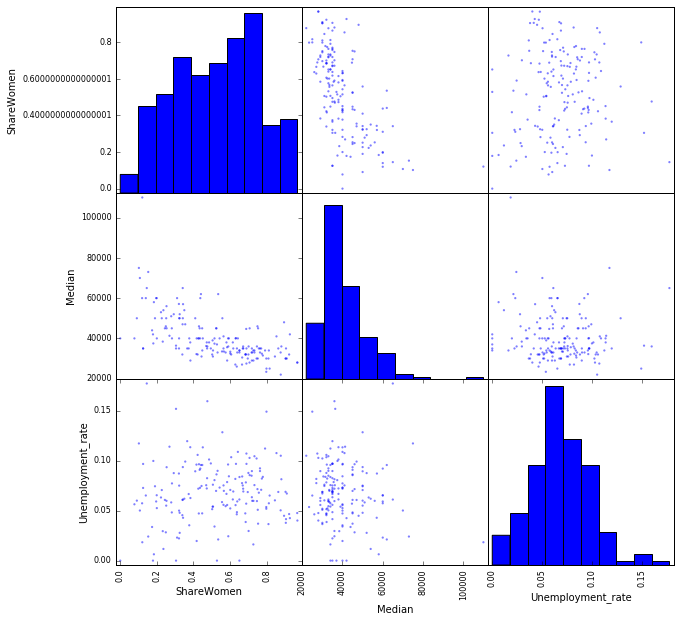In :
# a scatter matrix between 'ShareWomen' and'Employed':
pd.plotting.scatter_matrix(recent_grads[['ShareWomen', 'Employed']], figsize = (10, 10))
print ('The correlation value between the attributes are:\n {}' .format(recent_grads[['ShareWomen', 'Employed']].corr()))

The correlation value between the attributes are:
ShareWomen  Employed
ShareWomen    1.000000  0.147547
Employed      0.147547  1.000000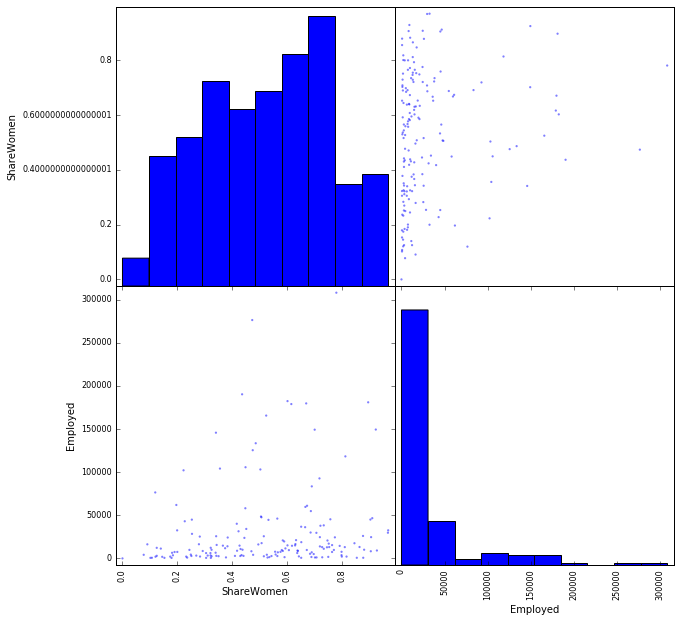### 4. Using bar plots to find the correlation between dataset attributes:¶

In :
# Comparing the percentages of women in the first 10 and last 10 rows of our dataset:
plt.show()
print('The mean value of columns :{}' .format(recent_grads[:10]['ShareWomen'].mean()))
plt.show()
print('The mean value of columns :{}' .format(recent_grads[-10:]['ShareWomen'].mean()))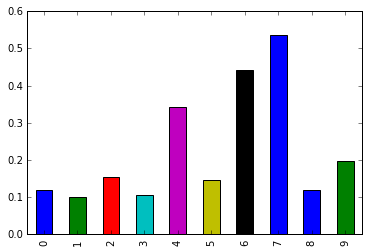The mean value of columns :0.226244326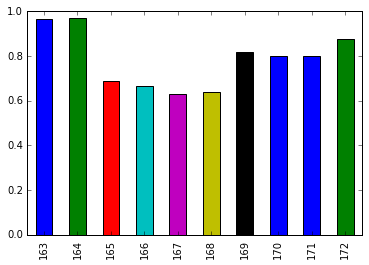The mean value of columns :0.7853643873999999


We see that the percentage of women increases as we move down the rows of the dataset.

In :
# Comparing the unemployment rate in the first 10 and last 10 rows of our dataset:
plt.show()
print('The mean value of columns :{}' .format(recent_grads[:10]['Unemployment_rate'].mean()))
plt.show()
print('The mean value of columns :{}' .format(recent_grads[-10:]['Unemployment_rate'].mean()))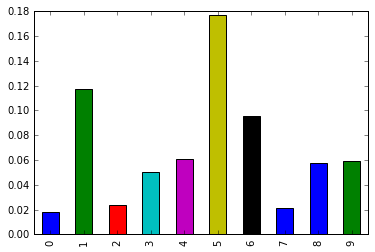The mean value of columns :0.06815034360000001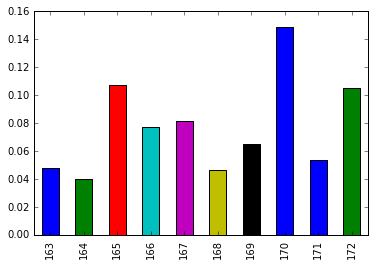The mean value of columns :0.0773135073


We see that the unemployment rate also increases as we move down the rows of the dataset.

Using the plots and values from above let's finally answer the questions that we sought to draw from the dataset:

1. Do students in more popular majors make more money? : Using the scatter plots, we see that for small sample_size the range of median salary was higher, indicating that students in less popular majors make more money.

2. Do students that majored in subjects that were majority female make more money? : Using the women vs median scatter plot we find subjects that had more females provide a lesser median salary.

3. Is there any link between the number of full-time employees and median salary?: From the scatter plot we found that lesser the number of full-time employees, higher is the range of the salary.

Let's if we can verify our findings numerically:

In :
# Let's find the mean of the median salaries of the 10 most popular and 10 least popular majors:
med = 0;
for val in top_tot:
total_pop = row['Median']
med = med + int(total_pop)

print ('The mean of median salaries of the top 10 most popular majors is: {}'
.format(med/len(top_tot)))

med = 0;
for val in low_tot:
total_pop = row['Median']
med = med + int(total_pop)

print ('The mean of median salaries of the 10 least popular majors is: {}'
.format(med/len(low_tot)))

The mean of median salaries of the top 10 most popular majors is: 37890.0
The mean of median salaries of the 10 least popular majors is: 46600.0


Hence can conclusively prove that students from the lesser popular majors earn more than that of the popular ones.

In :
# Let's find all the majors more populated with women and find the mean of their median salaries:

Out:
34605.208333333336
In :
# Let's compare our findings to the majors populated with men:

Out:
46988.15789473684

We therefore see that majors that had more women, provided a lesser median salary on average.

In :
# Let's find the mean of the median salaries of the 10 majors with most full_time employees to the least ones:
med = 0;
for val in top_tot:
total_pop = row['Median']
med = med + int(total_pop)

print ('The mean of median salaries of the majors with most full time emloyees: {}'
.format(med/len(top_tot)))

med = 0;
for val in low_tot:
total_pop = row['Median']
med = med + int(total_pop)

print ('The mean of median salaries of the 10 majors with least full time employees: {}'
.format(med/len(low_tot)))

The mean of median salaries of the majors with most full time emloyees: 38790.0
The mean of median salaries of the 10 majors with least full time employees: 45700.0


Hence we see that lesser the number of full_time employees, more the median salary on average.

In :
# grouped bar plot to compare the number of men with the number of women in each category of majors.

Out:
<matplotlib.axes._subplots.AxesSubplot at 0x7f4b8e7514e0>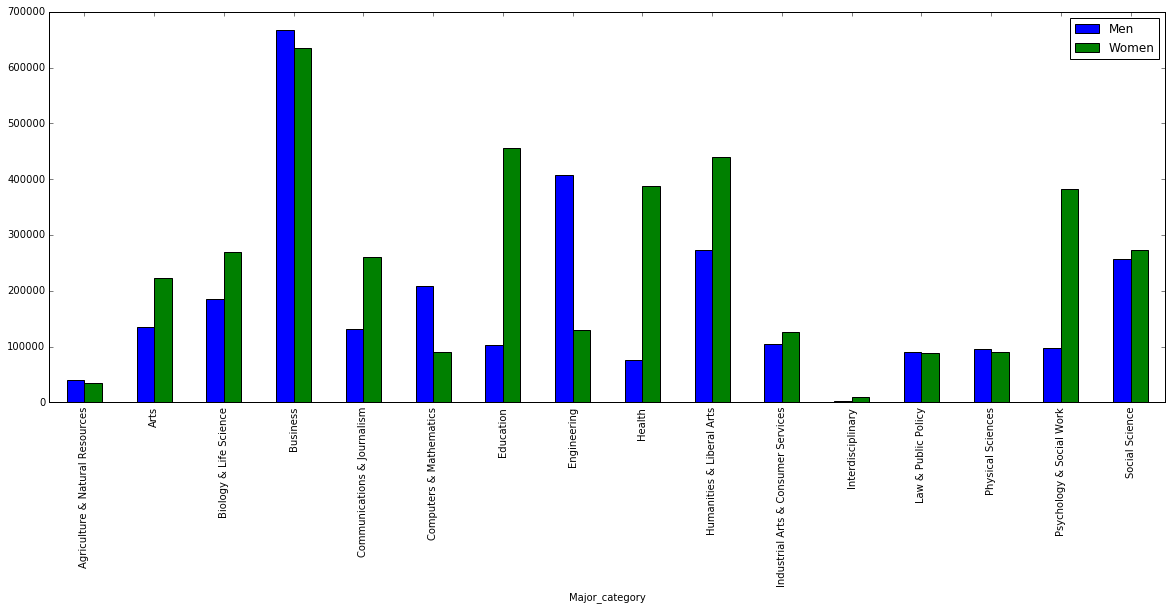Observations :

• Most popular major category : Business
• Male dominated majors: Engineering,Computers and Mathematics
• Female dominated majors : Arts, Biology & Life Sciences, Communications and Journalism, Education, Health, Humanities and Liberal Arts, Psychology and Social Work.
• Equally populated majors : Agriculture & Natural Resources, Business, Industrial Arts & Consumer Services, Law & Public Policy, Physical Sciences, Physical Sciences and Social Science
• Least popular major category : Interdisciplinary (:combination of categories), otherwise : Communications & Journalism
In :
#  box plot to explore the distributions of median salaries and unemployment rate:
plt.show()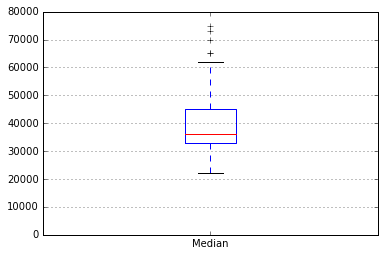In :
recent_grads['Unemployment_rate'].plot(kind='box').grid(axis='y')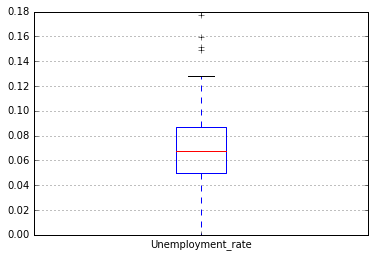Observations :

• Least median salary is in the lower 20's range.
• 50% of the salaries are between 30K-45K.
• 50% of the unemployment range is between 0.05-0.09 (i.e 5% - 9% of the total students enrolled in the majors.)
• 75% of the majors have unemployment rate below 9 percent
In :
# Let's see if we can numerically verify our findings:

Out:
Median Unemployment_rate
count 172.000000 172.000000
mean 40076.744186 0.068024
std 11461.388773 0.030340
min 22000.000000 0.000000
25% 33000.000000 0.050261
50% 36000.000000 0.067544
75% 45000.000000 0.087247
max 110000.000000 0.177226

## Conclusion:¶

1. On average, less popular majors provide higher salaries.
2. On average, the umemployment rate is 6.8%.
3. Men have higher salaries than women, indicating a high income disparity.
4. Branches like Engineering are still male dominant, whereas those like Health and Education are female dominant.

In [ ]: